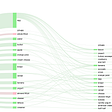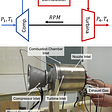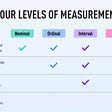# Selecting the right statistical test for our requirement.

What is the purpose of doing the statistical test?

A statistical test provides a mechanism for making a quantitative decision about the process and it allows us to make sense and interpret a great deal of information and it gives numerical evidence to draw valid conclusions from the test results. Using statistical analysis, we can determine the likelihood that a hypothesis should be either accepted or rejected. Most statistical tests are conducted under the assumption that measurements in the underlying population follow some known distribution. The reason for doing a statistical test is to find solutions for predictive function-based data.

Steps involved in performing a statistical test:

1. Framing hypothesis.

2. Identification of statistical test.

3. Finding the test statistic (stats value) and probability value (p-value).

4. Interpreting the test results.

Framing hypothesis: — Assumptions are made without seeing the data. Types of hypothesis a) Null hypothesis(ho) b) Alternate hypothesis(ha/h1). Hypothesis testing provides a method to reject a null hypothesis within a certain confidence level. But the reason why we reject the null hypothesis is that, if we accept the null hypothesis the independent features do not have any influence on the prediction of the target variable. The alternative hypothesis proposes that there is a difference.

Identification of statistical test

This is the most important step to choose the right statistical test for our variables.

What is the significance level?

The default significance level (α) is 0.05 indicates a 5% risk of concluding that aims to quantify evidence against a particular hypothesis being true.

· If p value> α (accept Ho)

· If p value< α (reject Ho)

How do you find the normality of your data?

We can check with the help of Shapiro–Wilk test or Jarque-Bera Test. If the p-value is less than the significance level, then ho is rejected else ha/h1 is selected.

Certain assumptions are made while doing a non-parametric test. The non-parametric test is done when data is not normally distributed.

Ho: skew=0 (or) p value> significance level(Data is normal)

Ha(or) h1: skew!=0 (or) p value≤ significance level (Data is not normal)

Interpreting the test results

If Alternate Hypotheses are selected then it will be useful in predicting the output.

Ha H1: variable_1(mu1) != variable_2(mu2)

(or)

p-value ≤ significance level

If a Null Hypothesis is selected then it will not be useful in predicting the output

Ho: variable_1(mu1) = variable_2(mu2)

(or)

p-value > significance level

Footnotes

Yes, it’s important to do statistical analysis before jumping into the Machine learning algorithms.

Hope you gained some useful insights.

And,💙 if this was a good read. Enjoy!

--

--

--

## More from Analytics Vidhya

Analytics Vidhya is a community of Analytics and Data Science professionals. We are building the next-gen data science ecosystem https://www.analyticsvidhya.com

## The Design And Research Of Vertical Search Engine## Analyzing GCS Respondent-Level Data with Python — First Steps## Part 3 Typeless Search: Discoverability## Write Better Stories with this Python Tool## Practical-1 |Practical-2 | Practical-3 | Practical-4 | Practical-5 | Practical-6 | Practical-7 |…## Practical example on data science and aeronautics## IBM Developer Day — Data Science, Machine Learning and AI## The Size of The Scuba Diving Industry## Lakshmi Sruthi

Aspiring Data Scientist

## Sample Variance: How does n-1 come?## Non-Parametric Test in Statistics## Visiting Seattle — the data are in!## What is data and why is it important? layman explanation | Data | Importance | type of data